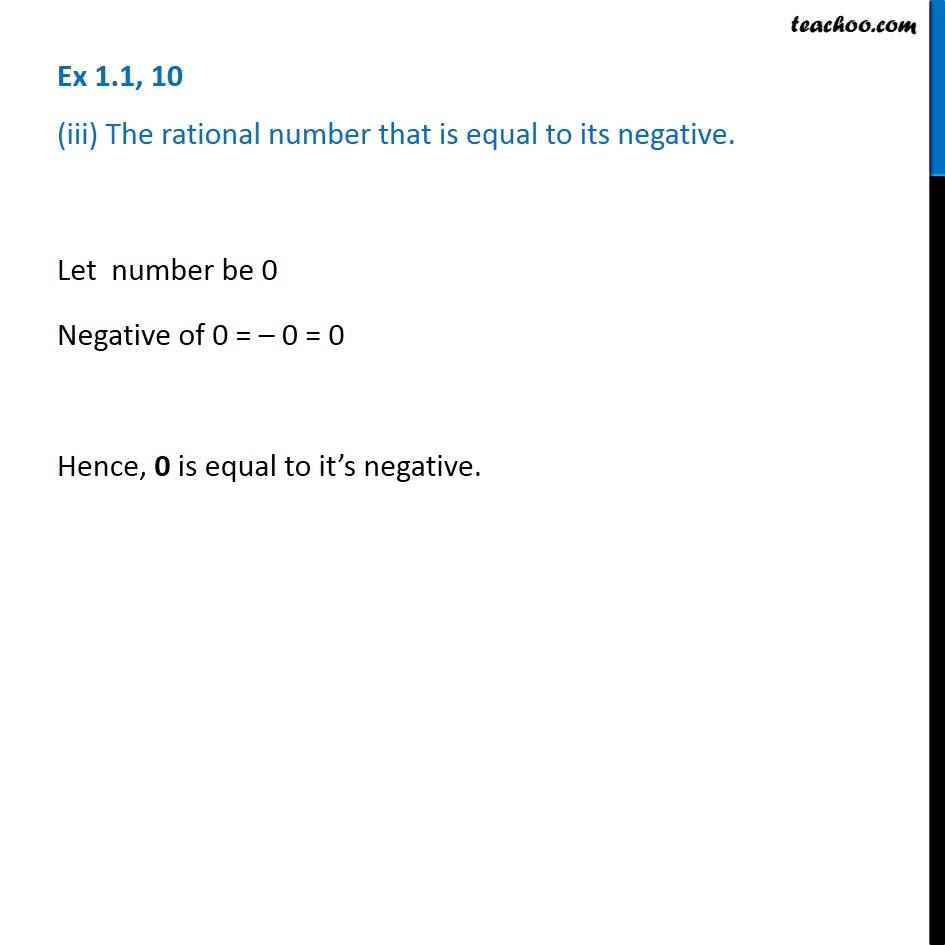1. Chapter 1 Class 8 Rational Numbers
2. Serial order wise
3. Ex 1.1

Transcript

Ex 1.1, 10 (iii) The rational number that is equal to its negative. Let number be 0 Negative of 0 = 0 = 0 Hence, 0 is equal to it s negative.